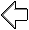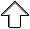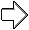OpenGL Programming Guide
Programming Guide > Chapter 12

## Chapter 12 Evaluators and NURBS

Chapter Objectives

After reading this chapter, you'll be able to do the following:

• Use OpenGL evaluator commands to draw basic curves and surfaces
• Use the GLU's higher-level NURBS facility to draw more complex curves and surfaces

Note that this chapter presumes a number of prerequisites; they're listed in "Prerequisites."

At the lowest level, graphics hardware draws points, line segments, and polygons, which are usually triangles and quadrilaterals. Smooth curves and surfaces are drawn by approximating them with large numbers of small line segments or polygons. However, many useful curves and surfaces can be described mathematically by a small number of parameters such as a few control points. Saving the 16 control points for a surface requires much less storage than saving 1000 triangles together with the normal vector information at each vertex. In addition, the 1000 triangles only approximate the true surface, but the control points accurately describe the real surface.

Evaluators provide a way to specify points on a curve or surface (or part of one) using only the control points. The curve or surface can then be rendered at any precision. In addition, normal vectors can be calculated for surfaces automatically. You can use the points generated by an evaluator in many ways - to draw dots where the surface would be, to draw a wireframe version of the surface, or to draw a fully lighted, shaded, and even textured version.

You can use evaluators to describe any polynomial or rational polynomial splines or surfaces of any degree. These include almost all splines and spline surfaces in use today, including B-splines, NURBS (Non-Uniform Rational B-Spline) surfaces, Bezier curves and surfaces, and Hermite splines. Since evaluators provide only a low-level description of the points on a curve or surface, they're typically used underneath utility libraries that provide a higher-level interface to the programmer. The GLU's NURBS facility is such a higher-level interface - the NURBS routines encapsulate lots of complicated code. Much of the final rendering is done with evaluators, but for some conditions (trimming curves, for example) the NURBS routines use planar polygons for rendering.

This chapter contains the following major sections.

• "Prerequisites" discusses what knowledge is assumed for this chapter. It also gives several references where you can obtain this information.
• "Evaluators" explains how evaluators work and how to control them using the appropriate OpenGL commands.
• "The GLU NURBS Interface" describes the GLU routines for creating NURBS surfaces.

## Prerequisites

Evaluators make splines and surfaces that are based on a Bezier (or Bernstein) basis. The defining formulas for the functions in this basis are given in this chapter, but the discussion doesn't include derivations or even lists of all their interesting mathematical properties. If you want to use evaluators to draw curves and surfaces using other bases, you must know how to convert your basis to a Bezier basis. In addition, when you render a Bezier surface or part of it using evaluators, you need to determine the granularity of your subdivision. Your decision needs to take into account the trade-off between high-quality (highly subdivided) images and high speed. Determining an appropriate subdivision strategy can be quite complicated - too complicated to be discussed here.

Similarly, a complete discussion of NURBS is beyond the scope of this book. The GLU NURBS interface is documented here, and programming examples are provided for readers who already understand the subject. In what follows, you already should know about NURBS control points, knot sequences, and trimming curves.

If you lack some of these prerequisites, the following references will help.

• Farin, Gerald E., Curves and Surfaces for Computer-Aided Geometric Design, Fourth Edition. San Diego, CA: Academic Press, 1996.
• Farin, Gerald E., NURB Curves and Surfaces: from Projective Geometry to Practical Use. Wellesley, MA: A. K. Peters Ltd., 1995.
• Farin, Gerald E., editor, NURBS for Curve and Surface Design, Society for Industrial and Applied Mathematics, Philadelphia, PA, 1991.
• Hoschek, Josef and Dieter Lasser, Fundamentals of Computer Aided Geometric Design. Wellesley, MA: A. K. Peters Ltd., 1993.
• Piegl, Les and Wayne Tiller, The NURBS Book. New York, NY: Springer-Verlag, 1995.

Note: Some terms used in this chapter might have slightly different meanings in other books on spline curves and surfaces, since there isn't total agreement among the practitioners of this art. Generally, the OpenGL meanings are a bit more restrictive. For example, OpenGL evaluators always use Bezier bases; in other contexts, evaluators might refer to the same concept, but with an arbitrary basis.

## Evaluators

A Bezier curve is a vector-valued function of one variable

C(u) = [X(u) Y(u) Z(u)]

where u varies in some domain (say [0,1]). A Bezier surface patch is a vector-valued function of two variables

S(u,v) = [X(u,v) Y(u,v) Z(u,v)]

where u and v can both vary in some domain. The range isn't necessarily three-dimensional as shown here. You might want two-dimensional output for curves on a plane or texture coordinates, or you might want four-dimensional output to specify RGBA information. Even one-dimensional output may make sense for gray levels.

For each u (or u and v, in the case of a surface), the formula for C() (or S()) calculates a point on the curve (or surface). To use an evaluator, first define the function C() or S(), enable it, and then use the glEvalCoord1() or glEvalCoord2() command instead of glVertex*(). This way, the curve or surface vertices can be used like any other vertices - to form points or lines, for example. In addition, other commands automatically generate series of vertices that produce a regular mesh uniformly spaced in u (or in u and v). One- and two-dimensional evaluators are similar, but the description is somewhat simpler in one dimension, so that case is discussed first.

### One-Dimensional Evaluators

This section presents an example of using one-dimensional evaluators to draw a curve. It then describes the commands and equations that control evaluators.

#### One-Dimensional Example: A Simple Bezier Curve

The program shown in Example 12-1 draws a cubic Bezier curve using four control points, as shown in Figure 12-1.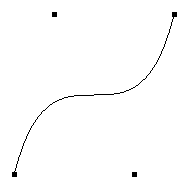Figure 12-1 : Bezier Curve

Example 12-1 : Bezier Curve with Four Control Points: bezcurve.c

```#include <GL/gl.h>
#include <GL/glu.h>
#include <stdlib.h>
#include <GL/glut.h>

GLfloat ctrlpoints = {
{ -4.0, -4.0, 0.0}, { -2.0, 4.0, 0.0},
{2.0, -4.0, 0.0}, {4.0, 4.0, 0.0}};

void init(void)
{
glClearColor(0.0, 0.0, 0.0, 0.0);
glMap1f(GL_MAP1_VERTEX_3, 0.0, 1.0, 3, 4, &ctrlpoints);
glEnable(GL_MAP1_VERTEX_3);
}

void display(void)
{
int i;

glClear(GL_COLOR_BUFFER_BIT);
glColor3f(1.0, 1.0, 1.0);
glBegin(GL_LINE_STRIP);
for (i = 0; i <= 30; i++)
glEvalCoord1f((GLfloat) i/30.0);
glEnd();
/* The following code displays the control points as dots. */
glPointSize(5.0);
glColor3f(1.0, 1.0, 0.0);
glBegin(GL_POINTS);
for (i = 0; i < 4; i++)
glVertex3fv(&ctrlpoints[i]);
glEnd();
glFlush();
}

void reshape(int w, int h)
{
glViewport(0, 0, (GLsizei) w, (GLsizei) h);
glMatrixMode(GL_PROJECTION);
if (w <= h)
glOrtho(-5.0, 5.0, -5.0*(GLfloat)h/(GLfloat)w,
5.0*(GLfloat)h/(GLfloat)w, -5.0, 5.0);
else
glOrtho(-5.0*(GLfloat)w/(GLfloat)h,
5.0*(GLfloat)w/(GLfloat)h, -5.0, 5.0, -5.0, 5.0);
glMatrixMode(GL_MODELVIEW);
}

int main(int argc, char** argv)
{
glutInit(&argc, argv);
glutInitDisplayMode (GLUT_SINGLE | GLUT_RGB);
glutInitWindowSize (500, 500);
glutInitWindowPosition (100, 100);
glutCreateWindow (argv);
init ();
glutDisplayFunc(display);
glutReshapeFunc(reshape);
glutMainLoop();
return 0;
}
```

A cubic Bezier curve is described by four control points, which appear in this example in the ctrlpoints[][] array. This array is one of the arguments to glMap1f(). All the arguments for this command are as follows:

GL_MAP1_VERTEX_3 Three-dimensional control points are provided and three-dimensional vertices are produced
0.0 Low value of parameter u
1.0 High value of parameter u
3 The number of floating-point values to advance in the data between one control point and the next
4 The order of the spline, which is the degree+1: in this case, the degree is 3 (since this is a cubic curve)
&ctrlpoints Pointer to the first control point's data

Note that the second and third arguments control the parameterization of the curve - as the variable u ranges from 0.0 to 1.0, the curve goes from one end to the other. The call to glEnable() enables the one-dimensional evaluator for three-dimensional vertices.

The curve is drawn in the routine display() between the glBegin() and glEnd() calls. Since the evaluator is enabled, the command glEvalCoord1f() is just like issuing a glVertex() command with the coordinates of a vertex on the curve corresponding to the input parameter u.

#### Defining and Evaluating a One-Dimensional Evaluator

The Bernstein polynomial of degree n (or order n+1) is given by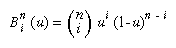If Pi represents a set of control points (one-, two-, three-, or even four- dimensional), then the equation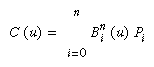represents a Bezier curve as u varies from 0.0 to 1.0. To represent the same curve but allowing u to vary between u1 and u2 instead of 0.0 and 1.0, evaluate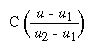The command glMap1() defines a one-dimensional evaluator that uses these equations.

void glMap1{fd}(GLenum target, TYPEu1, TYPEu2, GLint stride,
GLint
order, const TYPE*points);
Defines a one-dimensional evaluator. The target parameter specifies what the control points represent, as shown in Table 12-1, and therefore how many values need to be supplied in points. The points can represent vertices, RGBA color data, normal vectors, or texture coordinates. For
example, with GL_MAP1_COLOR_4, the evaluator generates color data along a curve in four-dimensional (RGBA) color space. You also use the parameter values listed in Table 12-1 to enable each defined evaluator before you invoke it. Pass the appropriate value to glEnable() or glDisable() to enable or disable the evaluator.
The second two parameters for glMap1*(), u1 and u2, indicate the range for the variable u. The variable stride is the number of single- or double-precision values (as appropriate) in each block of storage. Thus, it's an offset value between the beginning of one control point and the beginning of the next.
The order is the degree plus one, and it should agree with the number of control points. The points parameter points to the first coordinate of the first control point. Using the example data structure for glMap1*(), use the following for points:
```(GLfloat *)(&ctlpoints.x)

Table 12-1 : Types of Control Points for glMap1*()```
 Parameter Meaning GL_MAP1_VERTEX_3 x, y, z vertex coordinates GL_MAP1_VERTEX_4 x, y, z, w vertex coordinates GL_MAP1_INDEX color index GL_MAP1_COLOR_4 R, G, B, A GL_MAP1_NORMAL normal coordinates GL_MAP1_TEXTURE_COORD_1 s texture coordinates GL_MAP1_TEXTURE_COORD_2 s, t texture coordinates GL_MAP1_TEXTURE_COORD_3 s, t, r texture coordinates GL_MAP1_TEXTURE_COORD_4 s, t, r, q texture coordinates

More than one evaluator can be evaluated at a time. If you have both a GL_MAP1_VERTEX_3 and a GL_MAP1_COLOR_4 evaluator defined and enabled, for example, then calls to glEvalCoord1() generate both a position and a color. Only one of the vertex evaluators can be enabled at a time, although you might have defined both of them. Similarly, only one of the texture evaluators can be active. Other than that, however, evaluators can be used to generate any combination of vertex, normal, color, and texture-coordinate data. If more than one evaluator of the same type is defined and enabled, the one of highest dimension is used.

Use glEvalCoord1*() to evaluate a defined and enabled one-dimensional map.

void glEvalCoord1{fd}(TYPE u);
void glEvalCoord1{fd}v(
TYPE *u);
Causes evaluation of the enabled one-dimensional maps. The argument u is the value (or a pointer to the value, in the vector version of the command) of the domain coordinate.

For evaluated vertices, values for color, color index, normal vectors, and texture coordinates are generated by evaluation. Calls to glEvalCoord*() do not use the current values for color, color index, normal vectors, and texture coordinates. glEvalCoord*() also leaves those values unchanged.

#### Defining Evenly Spaced Coordinate Values in One Dimension

You can use glEvalCoord1() with any values for u, but by far the most common use is with evenly spaced values, as shown previously in Example 12-1. To obtain evenly spaced values, define a one-dimensional grid using glMapGrid1*() and then apply it using glEvalMesh1().

void glMapGrid1{fd}(GLint n, TYPEu1, TYPEu2);
Defines a grid that goes from u1 to u2 in n steps, which are evenly spaced.
void glEvalMesh1(GLenum mode, GLint p1, GLint p2);
Applies the currently defined map grid to all enabled evaluators. The mode can be either GL_POINT or GL_LINE, depending on whether you want to draw points or a connected line along the curve. The call has exactly the same effect as issuing a glEvalCoord1() for each of the steps between and including p1 and p2, where 0 <= p1, p2 <= n. Programmatically, it's equivalent to the following:
```glBegin(GL_POINTS);    /* OR glBegin(GL_LINE_STRIP); */
for (i = p1; i <= p2; i++)
glEvalCoord1(u1 + i*(u2-u1)/n);
glEnd();```
except that if i = 0 or i = n, then glEvalCoord1() is called with exactly u1 or u2 as its parameter.

### Two-Dimensional Evaluators

In two dimensions, everything is similar to the one-dimensional case, except that all the commands must take two parameters, u and v, into account. Points, colors, normals, or texture coordinates must be supplied over a surface instead of a curve. Mathematically, the definition of a Bezier surface patch is given by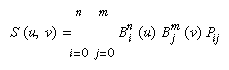where Pij are a set of m*n control points, and the Bi are the same Bernstein polynomials for one dimension. As before, the Pij can represent vertices, normals, colors, or texture coordinates.

The procedure to use two-dimensional evaluators is similar to the procedure for one dimension.

Define the evaluator(s) with glMap2*().

Enable them by passing the appropriate value to glEnable().

Invoke them either by calling glEvalCoord2() between a glBegin() and glEnd() pair or by specifying and then applying a mesh with glMapGrid2() and glEvalMesh2().

#### Defining and Evaluating a Two-Dimensional Evaluator

Use glMap2*() and glEvalCoord2*() to define and then invoke a two-dimensional evaluator.

void glMap2{fd}(GLenum target, TYPEu1, TYPEu2, GLint ustride,
GLint
uorder, TYPEv1, TYPEv2, GLint vstride,
GLint
vorder, TYPE points);
The target parameter can have any of the values in Table 12-1, except that the string MAP1 is replaced with MAP2. As before, these values are also used with glEnable() to enable the corresponding evaluator. Minimum and maximum values for both u and v are provided as u1, u2, v1, and v2. The parameters ustride and vstride indicate the number of single- or double-precision values (as appropriate) between independent settings for these values, allowing users to select a subrectangle of control points out of a much larger array. For example, if the data appears in the form
`GLfloat ctlpoints;`

and you want to use the 4x4 subset beginning at ctlpoints, choose
ustride to be 100*3 and vstride to be 3. The starting point, points, should be set to &ctlpoints. Finally, the order parameters, uorder and vorder, can be different, allowing patches that are cubic in one direction and quadratic in the other, for example.
void glEvalCoord2{fd}(TYPE u, TYPE v);
void glEvalCoord2{fd}v(
TYPE *values);
Causes evaluation of the enabled two-dimensional maps. The arguments u and v are the values (or a pointer to the values u and v, in the vector version of the command) for the domain coordinates. If either of the vertex evaluators is enabled (GL_MAP2_VERTEX_3 or GL_MAP2_VERTEX_4), then the normal to the surface is computed analytically. This normal is associated with the generated vertex if automatic normal generation has been enabled by passing GL_AUTO_NORMAL to glEnable(). If it's disabled, the corresponding enabled normal map is used to produce a normal. If no such map exists, the current normal is used.

#### Two-Dimensional Example: A Bezier Surface

Example 12-2 draws a wireframe Bezier surface using evaluators, as shown in Figure 12-2. In this example, the surface is drawn with nine curved lines in each direction. Each curve is drawn as 30 segments. To get the whole program, add the reshape() and main() routines from Example 12-1.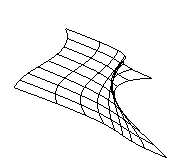Figure 12-2 : Bezier Surface

Example 12-2 : Bezier Surface: bezsurf.c

```#include <GL/gl.h>
#include <GL/glu.h>
#include <stdlib.h>
#include <GL/glut.h>

GLfloat ctrlpoints = {
{{-1.5, -1.5, 4.0}, {-0.5, -1.5, 2.0},
{0.5, -1.5, -1.0}, {1.5, -1.5, 2.0}},
{{-1.5, -0.5, 1.0}, {-0.5, -0.5, 3.0},
{0.5, -0.5, 0.0}, {1.5, -0.5, -1.0}},
{{-1.5, 0.5, 4.0}, {-0.5, 0.5, 0.0},
{0.5, 0.5, 3.0}, {1.5, 0.5, 4.0}},
{{-1.5, 1.5, -2.0}, {-0.5, 1.5, -2.0},
{0.5, 1.5, 0.0}, {1.5, 1.5, -1.0}}
};

void display(void)
{
int i, j;

glClear(GL_COLOR_BUFFER_BIT | GL_DEPTH_BUFFER_BIT);
glColor3f(1.0, 1.0, 1.0);
glPushMatrix ();
glRotatef(85.0, 1.0, 1.0, 1.0);
for (j = 0; j <= 8; j++) {
glBegin(GL_LINE_STRIP);
for (i = 0; i <= 30; i++)
glEvalCoord2f((GLfloat)i/30.0, (GLfloat)j/8.0);
glEnd();
glBegin(GL_LINE_STRIP);
for (i = 0; i <= 30; i++)
glEvalCoord2f((GLfloat)j/8.0, (GLfloat)i/30.0);
glEnd();
}
glPopMatrix ();
glFlush();
}

void init(void)
{
glClearColor (0.0, 0.0, 0.0, 0.0);
glMap2f(GL_MAP2_VERTEX_3, 0, 1, 3, 4,
0, 1, 12, 4, &ctrlpoints);
glEnable(GL_MAP2_VERTEX_3);
glMapGrid2f(20, 0.0, 1.0, 20, 0.0, 1.0);
glEnable(GL_DEPTH_TEST);
}```

#### Defining Evenly Spaced Coordinate Values in Two Dimensions

In two dimensions, the glMapGrid2*() and glEvalMesh2() commands are similar to the one-dimensional versions, except that both u and v information must be included.

void glMapGrid2{fd}(GLint nu, TYPEu1, TYPEu2,
GLint
nv, TYPEv1, TYPEv2);
void glEvalMesh2(GLenum
mode, GLint i1, GLint i2, GLint j1, GLint j2);
Defines a two-dimensional map grid that goes from u1 to u2 in nu evenly spaced steps, from v1 to v2 in nv steps (glMapGrid2*()), and then applies this grid to all enabled evaluators (glEvalMesh2()). The only significant difference from the one-dimensional versions of these two commands is that in glEvalMesh2() the mode parameter can be GL_FILL as well as GL_POINT or GL_LINE. GL_FILL generates filled polygons using the quad-mesh primitive. Stated precisely, glEvalMesh2() is nearly equivalent to one of the following three code fragments. (It's nearly equivalent because when i is equal to nu or j to nv, the parameter is exactly equal to u2 or v2, not to u1+nu*(u2-u1)/nu, which might be slightly different due to round-off error.)
```glBegin(GL_POINTS);                /* mode == GL_POINT */
for (i = nu1; i <= nu2; i++)
for (j = nv1; j <= nv2; j++)
glEvalCoord2(u1 + i*(u2-u1)/nu, v1+j*(v2-v1)/nv);
glEnd();```

or

```for (i = nu1; i <= nu2; i++) {     /* mode == GL_LINE */
glBegin(GL_LINES);
for (j = nv1; j <= nv2; j++)
glEvalCoord2(u1 + i*(u2-u1)/nu, v1+j*(v2-v1)/nv);
glEnd();
}
for (j = nv1; j <= nv2; j++) {
glBegin(GL_LINES);
for (i = nu1; i <= nu2; i++)
glEvalCoord2(u1 + i*(u2-u1)/nu, v1+j*(v2-v1)/nv);
glEnd();
}```

or

```for (i = nu1; i < nu2; i++) {     /* mode == GL_FILL */
for (j = nv1; j <= nv2; j++) {
glEvalCoord2(u1 + i*(u2-u1)/nu, v1+j*(v2-v1)/nv);
glEvalCoord2(u1 + (i+1)*(u2-u1)/nu, v1+j*(v2-v1)/nv);
glEnd();
}```

Example 12-3 shows the differences necessary to draw the same Bezier surface as Example 12-2, but using glMapGrid2() and glEvalMesh2() to subdivide the square domain into a uniform 8x8 grid. This program also adds lighting and shading, as shown in Figure 12-3.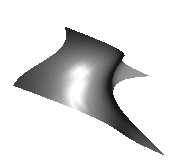Figure 12-3 : Lit, Shaded Bezier Surface Drawn with a Mesh

Example 12-3 : Lit, Shaded Bezier Surface Using a Mesh: bezmesh.c

```void initlights(void)
{
GLfloat ambient[] = {0.2, 0.2, 0.2, 1.0};
GLfloat position[] = {0.0, 0.0, 2.0, 1.0};
GLfloat mat_diffuse[] = {0.6, 0.6, 0.6, 1.0};
GLfloat mat_specular[] = {1.0, 1.0, 1.0, 1.0};
GLfloat mat_shininess[] = {50.0};

glEnable(GL_LIGHTING);
glEnable(GL_LIGHT0);

glLightfv(GL_LIGHT0, GL_AMBIENT, ambient);
glLightfv(GL_LIGHT0, GL_POSITION, position);

glMaterialfv(GL_FRONT, GL_DIFFUSE, mat_diffuse);
glMaterialfv(GL_FRONT, GL_SPECULAR, mat_specular);
glMaterialfv(GL_FRONT, GL_SHININESS, mat_shininess);
}

void display(void)
{
glClear(GL_COLOR_BUFFER_BIT | GL_DEPTH_BUFFER_BIT);
glPushMatrix();
glRotatef(85.0, 1.0, 1.0, 1.0);
glEvalMesh2(GL_FILL, 0, 20, 0, 20);
glPopMatrix();
glFlush();
}

void init(void)
{
glClearColor(0.0, 0.0, 0.0, 0.0);
glEnable(GL_DEPTH_TEST);
glMap2f(GL_MAP2_VERTEX_3, 0, 1, 3, 4,
0, 1, 12, 4, &ctrlpoints);
glEnable(GL_MAP2_VERTEX_3);
glEnable(GL_AUTO_NORMAL);
glMapGrid2f(20, 0.0, 1.0, 20, 0.0, 1.0);
initlights();
}```

### Using Evaluators for Textures

Example 12-4 enables two evaluators at the same time: The first generates three-dimensional points on the same Bezier surface as Example 12-3, and the second generates texture coordinates. In this case, the texture coordinates are the same as the u and v coordinates of the surface, but a special flat Bezier patch must be created to do this.

The flat patch is defined over a square with corners at (0, 0), (0, 1), (1, 0), and (1, 1); it generates (0, 0) at corner (0, 0), (0, 1) at corner (0, 1), and so on. Since it's of order two (linear degree plus one), evaluating this texture at the point (u, v) generates texture coordinates (s, t). It's enabled at the same time as the vertex evaluator, so both take effect when the surface is drawn. (See "Plate 19" in Appendix I.) If you want the texture to repeat three times in each direction, change every 1.0 in the array texpts[][][] to 3.0. Since the texture wraps in this example, the surface is rendered with nine copies of the texture map.

Example 12-4 : Using Evaluators for Textures: texturesurf.c

```#include <GL/gl.h>
#include <GL/glu.h>
#include <stdlib.h>
#include <GL/glut.h>
#include <math.h>

GLfloat ctrlpoints = {
{{ -1.5, -1.5, 4.0}, { -0.5, -1.5, 2.0},
{0.5, -1.5, -1.0}, {1.5, -1.5, 2.0}},
{{ -1.5, -0.5, 1.0}, { -0.5, -0.5, 3.0},
{0.5, -0.5, 0.0}, {1.5, -0.5, -1.0}},
{{ -1.5, 0.5, 4.0}, { -0.5, 0.5, 0.0},
{0.5, 0.5, 3.0}, {1.5, 0.5, 4.0}},
{{ -1.5, 1.5, -2.0}, { -0.5, 1.5, -2.0},
{0.5, 1.5, 0.0}, {1.5, 1.5, -1.0}}
};
GLfloat texpts = {{{0.0, 0.0}, {0.0, 1.0}},
{{1.0, 0.0}, {1.0, 1.0}}};

void display(void)
{
glClear(GL_COLOR_BUFFER_BIT | GL_DEPTH_BUFFER_BIT);
glColor3f(1.0, 1.0, 1.0);
glEvalMesh2(GL_FILL, 0, 20, 0, 20);
glFlush();
}
#define imageWidth 64
#define imageHeight 64
GLubyte image[3*imageWidth*imageHeight];

void makeImage(void)
{
int i, j;
float ti, tj;

for (i = 0; i < imageWidth; i++) {
ti = 2.0*3.14159265*i/imageWidth;
for (j = 0; j < imageHeight; j++) {
tj = 2.0*3.14159265*j/imageHeight;
image[3*(imageHeight*i+j)] =
(GLubyte) 127*(1.0+sin(ti));
image[3*(imageHeight*i+j)+1] =
(GLubyte) 127*(1.0+cos(2*tj));
image[3*(imageHeight*i+j)+2] =
(GLubyte) 127*(1.0+cos(ti+tj));
}
}
}

void init(void)
{
glMap2f(GL_MAP2_VERTEX_3, 0, 1, 3, 4,
0, 1, 12, 4, &ctrlpoints);
glMap2f(GL_MAP2_TEXTURE_COORD_2, 0, 1, 2, 2,
0, 1, 4, 2, &texpts);
glEnable(GL_MAP2_TEXTURE_COORD_2);
glEnable(GL_MAP2_VERTEX_3);
glMapGrid2f(20, 0.0, 1.0, 20, 0.0, 1.0);
makeImage();
glTexEnvf(GL_TEXTURE_ENV, GL_TEXTURE_ENV_MODE, GL_DECAL);
glTexParameteri(GL_TEXTURE_2D, GL_TEXTURE_WRAP_S, GL_REPEAT);
glTexParameteri(GL_TEXTURE_2D, GL_TEXTURE_WRAP_T, GL_REPEAT);
glTexParameteri(GL_TEXTURE_2D, GL_TEXTURE_MAG_FILTER,
GL_NEAREST);
glTexParameteri(GL_TEXTURE_2D, GL_TEXTURE_MIN_FILTER,
GL_NEAREST);
glTexImage2D(GL_TEXTURE_2D, 0, 3, imageWidth, imageHeight, 0,
GL_RGB, GL_UNSIGNED_BYTE, image);
glEnable(GL_TEXTURE_2D);
glEnable(GL_DEPTH_TEST);
}

void reshape(int w, int h)
{
glViewport(0, 0, (GLsizei) w, (GLsizei) h);
glMatrixMode(GL_PROJECTION);
if (w <= h)
glOrtho(-4.0, 4.0, -4.0*(GLfloat)h/(GLfloat)w,
4.0*(GLfloat)h/(GLfloat)w, -4.0, 4.0);
else
glOrtho(-4.0*(GLfloat)w/(GLfloat)h,
4.0*(GLfloat)w/(GLfloat)h, -4.0, 4.0, -4.0, 4.0);
glMatrixMode(GL_MODELVIEW);
glRotatef(85.0, 1.0, 1.0, 1.0);
}

int main(int argc, char** argv)
{
glutInit(&argc, argv);
glutInitDisplayMode (GLUT_SINGLE | GLUT_RGB | GLUT_DEPTH);
glutInitWindowSize (500, 500);
glutInitWindowPosition (100, 100);
glutCreateWindow (argv);
init ();
glutDisplayFunc(display);
glutReshapeFunc(reshape);
glutMainLoop();
return 0;
}```

## The GLU NURBS Interface

Although evaluators are the only OpenGL primitive available to draw curves and surfaces directly, and even though they can be implemented very efficiently in hardware, they're often accessed by applications through higher-level libraries. The GLU provides a NURBS (Non-Uniform Rational B-Spline) interface built on top of the OpenGL evaluator commands.

### A Simple NURBS Example

If you intend to use lighting with a NURBS surface, call glEnable() with GL_AUTO_NORMAL to automatically generate surface normals. (Or you can calculate your own.)

Use gluNewNurbsRenderer() to create a pointer to a NURBS object, which is referred to when creating your NURBS curve or surface.

If desired, call gluNurbsProperty() to choose rendering values, such as the maximum size of lines or polygons that are used to render your NURBS object.

Call gluNurbsCallback() if you want to be notified when an error is encountered. (Error checking may slightly degrade performance but is still highly recommended.)

Start your curve or surface by calling gluBeginCurve() or gluBeginSurface().

Generate and render your curve or surface. Call gluNurbsCurve() or gluNurbsSurface() at least once with the control points (rational or nonrational), knot sequence, and order of the polynomial basis function for your NURBS object. You might call these functions additional times to specify surface normals and/or texture coordinates.

Call gluEndCurve() or gluEndSurface() to complete the curve or surface.

Example 12-5 renders a NURBS surface in the shape of a symmetrical hill with control points ranging from -3.0 to 3.0. The basis function is a cubic B-spline, but the knot sequence is nonuniform, with a multiplicity of 4 at each endpoint, causing the basis function to behave like a Bezier curve in each direction. The surface is lighted, with a dark gray diffuse reflection and white specular highlights. Figure 12-4 shows the surface as a lit wireframe.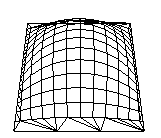Figure 12-4 : NURBS Surface

Example 12-5 : NURBS Surface: surface.c

```#include <GL/gl.h>
#include <GL/glu.h>
#include <GL/glut.h>
#include <stdlib.h>
#include <stdio.h>

GLfloat ctlpoints;
int showPoints = 0;

GLUnurbsObj *theNurb;

void init_surface(void)
{
int u, v;
for (u = 0; u < 4; u++) {
for (v = 0; v < 4; v++) {
ctlpoints[u][v] = 2.0*((GLfloat)u - 1.5);
ctlpoints[u][v] = 2.0*((GLfloat)v - 1.5);

if ( (u == 1 || u == 2) && (v == 1 || v == 2))
ctlpoints[u][v] = 3.0;
else
ctlpoints[u][v] = -3.0;
}
}
}

void nurbsError(GLenum errorCode)
{
const GLubyte *estring;

estring = gluErrorString(errorCode);
fprintf (stderr, "Nurbs Error: %s\n", estring);
exit (0);
}

void init(void)
{
GLfloat mat_diffuse[] = { 0.7, 0.7, 0.7, 1.0 };
GLfloat mat_specular[] = { 1.0, 1.0, 1.0, 1.0 };
GLfloat mat_shininess[] = { 100.0 };

glClearColor (0.0, 0.0, 0.0, 0.0);
glMaterialfv(GL_FRONT, GL_DIFFUSE, mat_diffuse);
glMaterialfv(GL_FRONT, GL_SPECULAR, mat_specular);
glMaterialfv(GL_FRONT, GL_SHININESS, mat_shininess);

glEnable(GL_LIGHTING);
glEnable(GL_LIGHT0);
glEnable(GL_DEPTH_TEST);
glEnable(GL_AUTO_NORMAL);
glEnable(GL_NORMALIZE);

init_surface();

theNurb = gluNewNurbsRenderer();
gluNurbsProperty(theNurb, GLU_SAMPLING_TOLERANCE, 25.0);
gluNurbsProperty(theNurb, GLU_DISPLAY_MODE, GLU_FILL);
gluNurbsCallback(theNurb, GLU_ERROR,
(GLvoid (*)()) nurbsError);
}

void display(void)
{
GLfloat knots = {0.0, 0.0, 0.0, 0.0, 1.0, 1.0, 1.0, 1.0};
int i, j;

glClear(GL_COLOR_BUFFER_BIT | GL_DEPTH_BUFFER_BIT);

glPushMatrix();
glRotatef(330.0, 1.,0.,0.);
glScalef (0.5, 0.5, 0.5);

gluBeginSurface(theNurb);
gluNurbsSurface(theNurb,
8, knots, 8, knots,
4 * 3, 3, &ctlpoints,
4, 4, GL_MAP2_VERTEX_3);
gluEndSurface(theNurb);

if (showPoints) {
glPointSize(5.0);
glDisable(GL_LIGHTING);
glColor3f(1.0, 1.0, 0.0);
glBegin(GL_POINTS);
for (i = 0; i < 4; i++) {
for (j = 0; j < 4; j++) {
glVertex3f(ctlpoints[i][j],
ctlpoints[i][j], ctlpoints[i][j]);
}
}
glEnd();
glEnable(GL_LIGHTING);
}
glPopMatrix();
glFlush();
}

void reshape(int w, int h)
{
glViewport(0, 0, (GLsizei) w, (GLsizei) h);
glMatrixMode(GL_PROJECTION);
gluPerspective (45.0, (GLdouble)w/(GLdouble)h, 3.0, 8.0);
glMatrixMode(GL_MODELVIEW);
glTranslatef (0.0, 0.0, -5.0);
}
void keyboard(unsigned char key, int x, int y)
{
switch (key) {
case `c':
case `C':
showPoints = !showPoints;
glutPostRedisplay();
break;
case 27:
exit(0);
break;
default:
break;
}
}

int main(int argc, char** argv)
{
glutInit(&argc, argv);
glutInitDisplayMode(GLUT_SINGLE | GLUT_RGB | GLUT_DEPTH);
glutInitWindowSize (500, 500);
glutInitWindowPosition (100, 100);
glutCreateWindow(argv);
init();
glutReshapeFunc(reshape);
glutDisplayFunc(display);
glutKeyboardFunc (keyboard);
glutMainLoop();
return 0;
}```

### Manage a NURBS Object

As shown in Example 12-5, gluNewNurbsRenderer() returns a new NURBS object, whose type is a pointer to a GLUnurbsObj structure. You must make this object before using any other NURBS routine. When you're done with a NURBS object, you may use gluDeleteNurbsRenderer() to free up the memory that was used.

GLUnurbsObj* gluNewNurbsRenderer (void);
Creates a new NURBS object, nobj. Returns a pointer to the new object, or zero, if OpenGL cannot allocate memory for a new NURBS object.
void gluDeleteNurbsRenderer (GLUnurbsObj *nobj);
Destroys the NURBS object nobj.

#### Control NURBS Rendering Properties

A set of properties associated with a NURBS object affects the way the object is rendered. These properties include how the surface is rasterized (for example, filled or wireframe) and the precision of tessellation.

void gluNurbsProperty(GLUnurbsObj *nobj, GLenum property,
GLfloat
value);
Controls attributes of a NURBS object, nobj. The property argument specifies the property and can be GLU_DISPLAY_MODE, GLU_CULLING, GLU_SAMPLING_METHOD, GLU_SAMPLING_TOLERANCE, GLU_PARAMETRIC_TOLERANCE, GLU_U_STEP, GLU_V_STEP, or GLU_AUTO_LOAD_MATRIX. The value argument indicates what the property should be.
The default value for GLU_DISPLAY_MODE is GLU_FILL, which causes the surface to be rendered as polygons. If GLU_OUTLINE_POLYGON is used for the display-mode property, only the outlines of polygons created by tessellation are rendered. GLU_OUTLINE_PATCH renders the outlines of patches and trimming curves. (See "Create a NURBS Curve or Surface".)
GLU_CULLING can speed up performance by not performing tessellation if the NURBS object falls completely outside the viewing volume; set this property to GL_TRUE to enable culling (the default is GL_FALSE).
Since a NURBS object is rendered as primitives, it's sampled at different values of its parameter(s) (u and v) and broken down into small line segments or polygons for rendering. If property is GLU_SAMPLING_METHOD, then value is set to one of GLU_PATH_LENGTH (which is the default), GLU_PARAMETRIC_ERROR, or GLU_DOMAIN_DISTANCE, which specifies how a NURBS curve or surface should be tessellated. When value is set to GLU_PATH_LENGTH, the surface is rendered so that the maximum length, in pixels, of the edges of tessellated polygons is no greater than what is specified by GLU_SAMPLING_TOLERANCE. When set to GLU_PARAMETRIC_ERROR, then the value specified by GLU_PARAMETRIC_TOLERANCE is the maximum distance, in pixels, between tessellated polygons and the surfaces they approximate. When set to GLU_DOMAIN_DISTANCE, the application specifies, in parametric coordinates, how many sample points per unit length are taken in the u and v dimensions, using the values for GLU_U_STEP and GLU_V_STEP.
If property is GLU_SAMPLING_TOLERANCE and the sampling method is GLU_PATH_LENGTH, value controls the maximum length, in pixels, to use for tessellated polygons. The default value of 50.0 makes the largest sampled line segment or polygon edge 50.0 pixels long. If property is GLU_PARAMETRIC_TOLERANCE and the sampling method is GLU_PARAMETRIC_ERROR, value controls the maximum distance, in pixels, between the tessellated polygons and the surfaces they approximate. The default value for GLU_PARAMETRIC_TOLERANCE is 0.5, which makes the tessellated polygons within one-half pixel of the approximated surface. If the sampling method is GLU_DOMAIN_DISTANCE and property is either GLU_U_STEP or GLU_V_STEP, then value is the number of sample points per unit length taken along the u or v dimension, respectively, in parametric coordinates. The default for both GLU_U_STEP and GLU_V_STEP is 100.
The GLU_AUTO_LOAD_MATRIX property determines whether the projection matrix, modelview matrix, and viewport are downloaded from the OpenGL server (GL_TRUE, the default), or whether the application must supply these matrices with gluLoadSamplingMatrices() (GL_FALSE).
void gluLoadSamplingMatrices (GLUnurbsObj *nobj, const GLfloat modelMatrix, const GLfloat projMatrix, const GLint viewport);
If the GLU_AUTO_LOAD_MATRIX is turned off, the modelview and projection matrices and the viewport specified in gluLoadSamplingMatrices() are used to compute sampling and culling matrices for each NURBS curve or surface.

If you need to query the current value for a NURBS property, you may use gluGetNurbsProperty().

void gluGetNurbsProperty (GLUnurbsObj *nobj, GLenum property,
GLfloat
*value);
Given the property to be queried for the NURBS object nobj, return its current value.

#### Handle NURBS Errors

Since there are 37 different errors specific to NURBS functions, it's a good idea to register an error callback to let you know if you've stumbled into one of them. In Example 12-5, the callback function was registered with

`gluNurbsCallback(theNurb, GLU_ERROR, (GLvoid (*)()) nurbsError);`
void gluNurbsCallback (GLUnurbsObj *nobj, GLenum which,
void (*
fn)(GLenum errorCode));
which is the type of callback; it must be GLU_ERROR. When a NURBS function detects an error condition, fn is invoked with the error code as its only argument. errorCode is one of 37 error conditions, named GLU_NURBS_ERROR1 through GLU_NURBS_ERROR37. Use gluErrorString() to describe the meaning of those error codes.

In Example 12-5, the nurbsError() routine was registered as the error callback function:

```void nurbsError(GLenum errorCode)
{
const GLubyte *estring;

estring = gluErrorString(errorCode);
fprintf (stderr, "Nurbs Error: %s\n", estring);
exit (0);
}```

### Create a NURBS Curve or Surface

To render a NURBS surface, gluNurbsSurface() is bracketed by gluBeginSurface() and gluEndSurface(). The bracketing routines save and restore the evaluator state.

void gluBeginSurface (GLUnurbsObj *nobj);
void gluEndSurface (GLUnurbsObj *nobj);
After gluBeginSurface(), one or more calls to gluNurbsSurface() defines the attributes of the surface. Exactly one of these calls must have a surface type of GL_MAP2_VERTEX_3 or GL_MAP2_VERTEX_4 to generate vertices. Use gluEndSurface() to end the definition of a surface. Trimming of NURBS surfaces is also supported between gluBeginSurface() and gluEndSurface(). (See "Trim a NURBS Surface".)
void gluNurbsSurface (GLUnurbsObj *nobj, GLint uknot_count,
GLfloat *
uknot, GLint vknot_count, GLfloat *vknot,
GLint
u_stride, GLint v_stride, GLfloat *ctlarray,
GLint
uorder, GLint vorder, GLenum type);
Describes the vertices (or surface normals or texture coordinates) of a NURBS surface, nobj. Several of the values must be specified for both u and v parametric directions, such as the knot sequences (uknot and vknot), knot counts (uknot_count and vknot_count), and order of the polynomial (uorder and vorder) for the NURBS surface. Note that the number of control points isn't specified. Instead, it's derived by determining the number of control points along each parameter as the number of knots minus the order. Then, the number of control points for the surface is equal to the number of control points in each parametric direction, multiplied by one another. The ctlarray argument points to an array of control points.
The last parameter, type, is one of the two-dimensional evaluator types. Commonly, you might use GL_MAP2_VERTEX_3 for nonrational or GL_MAP2_VERTEX_4 for rational control points, respectively. You might also use other types, such as GL_MAP2_TEXTURE_COORD_* or GL_MAP2_NORMAL to calculate and assign texture coordinates or surface normals. For example, to create a lighted (with surface normals) and textured NURBS surface, you may need to call this sequence:
```gluBeginSurface(nobj);
gluNurbsSurface(nobj, ..., GL_MAP2_TEXTURE_COORD_2);
gluNurbsSurface(nobj, ..., GL_MAP2_NORMAL);
gluNurbsSurface(nobj, ..., GL_MAP2_VERTEX_3);
gluEndSurface(nobj);```
The u_stride and v_stride arguments represent the number of floating-point values between control points in each parametric direction. The evaluator type, as well as its order, affects the u_stride and v_stride values. In Example 12-5, u_stride is 12 (4 * 3) because there are three coordinates for each vertex (set by GL_MAP2_VERTEX_3) and four control points in the parametric v direction; v_stride is 3 because each vertex had three coordinates, and v control points are adjacent to one another.

Drawing a NURBS curve is similar to drawing a surface, except that all calculations are done with one parameter, u, rather than two. Also, for curves, gluBeginCurve() and gluEndCurve() are the bracketing routines.

void gluBeginCurve (GLUnurbsObj *nobj);
void gluEndCurve (GLUnurbsObj
*nobj);
After gluBeginCurve(), one or more calls to gluNurbsCurve() define the attributes of the surface. Exactly one of these calls must have a surface type of GL_MAP1_VERTEX_3 or GL_MAP1_VERTEX_4 to generate vertices. Use gluEndCurve() to end the definition of a surface.
void gluNurbsCurve (GLUnurbsObj *nobj, GLint uknot_count,
GLfloat *
uknot, GLint u_stride, GLfloat *ctlarray,
GLint
uorder, GLenum type);
Defines a NURBS curve for the object nobj. The arguments have the same meaning as those for gluNurbsSurface(). Note that this routine requires only one knot sequence and one declaration of the order of the NURBS object. If this curve is defined within a gluBeginCurve()/gluEndCurve() pair, then the type can be any of the valid one-dimensional evaluator types (such as GL_MAP1_VERTEX_3 or GL_MAP1_VERTEX_4).

### Trim a NURBS Surface

To create a trimmed NURBS surface with OpenGL, start as if you were creating an untrimmed surface. After calling gluBeginSurface() and gluNurbsSurface() but before calling gluEndSurface(), start a trim by calling gluBeginTrim().

void gluBeginTrim (GLUnurbsObj *nobj);
void gluEndTrim (GLUnurbsObj *
nobj);
Marks the beginning and end of the definition of a trimming loop. A trimming loop is a set of oriented, trimming curve segments (forming a closed curve) that defines the boundaries of a NURBS surface.

You can create two kinds of trimming curves, a piecewise linear curve with gluPwlCurve() or a NURBS curve with gluNurbsCurve(). A piecewise linear curve doesn't look like what's conventionally called a curve, because it's a series of straight lines. A NURBS curve for trimming must lie within the unit square of parametric (u, v) space. The type for a NURBS trimming curve is usually GLU_MAP1_TRIM2. Less often, the type is GLU_MAP1_TRIM3, where the curve is described in a two-dimensional homogeneous space (u', v', w') by (u, v) = (u'/w', v'/w').

void gluPwlCurve (GLUnurbsObj *nobj, GLint count, GLfloat *array,
GLint
stride, GLenum type);
Describes a piecewise linear trimming curve for the NURBS object nobj. There are count points on the curve, and they're given by array. The type can be either GLU_MAP1_TRIM_2 (the most common) or GLU_MAP1_TRIM_3 ((u, v, w) homogeneous parameter space). The type affects whether stride, the number of floating-point values to the next vertex, is 2 or 3.

You need to consider the orientation of trimming curves - that is, whether they're counterclockwise or clockwise - to make sure you include the desired part of the surface. If you imagine walking along a curve, everything to the left is included and everything to the right is trimmed away. For example, if your trim consists of a single counterclockwise loop, everything inside the loop is included. If the trim consists of two nonintersecting counterclockwise loops with nonintersecting interiors, everything inside either of them is included. If it consists of a counterclockwise loop with two clockwise loops inside it, the trimming region has two holes in it. The outermost trimming curve must be counterclockwise. Often, you run a trimming curve around the entire unit square to include everything within it, which is what you get by default by not specifying any trimming curves.

Trimming curves must be closed and nonintersecting. You can combine trimming curves, so long as the endpoints of the trimming curves meet to form a closed curve. You can nest curves, creating islands that float in space. Be sure to get the curve orientations right. For example, an error results if you specify a trimming region with two counterclockwise curves, one enclosed within another: The region between the curves is to the left of one and to the right of the other, so it must be both included and excluded, which is impossible. Figure 12-5 illustrates a few valid possibilities.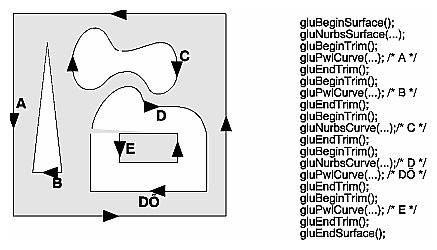Figure 12-5 : Parametric Trimming Curves

Figure 12-6 shows the same small hill as in Figure 12-4, this time with a trimming curve that's a combination of a piecewise linear curve and a NURBS curve. The program that creates this figure is similar to that shown in Example 12-5; the differences are in the routines shown in Example 12-6.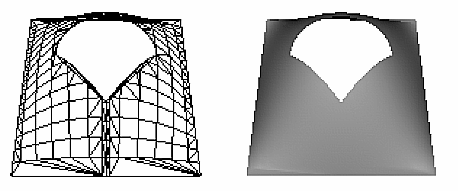Figure 12-6 : Trimmed NURBS Surface

Example 12-6 : Trimming a NURBS Surface: trim.c

```void display(void)
{
GLfloat knots = {0.0, 0.0, 0.0, 0.0, 1.0, 1.0, 1.0, 1.0};
GLfloat edgePt = /* counter clockwise */
{{0.0, 0.0}, {1.0, 0.0}, {1.0, 1.0}, {0.0, 1.0},
{0.0, 0.0}};
GLfloat curvePt = /* clockwise */
{{0.25, 0.5}, {0.25, 0.75}, {0.75, 0.75}, {0.75, 0.5}};
GLfloat curveKnots =
{0.0, 0.0, 0.0, 0.0, 1.0, 1.0, 1.0, 1.0};
GLfloat pwlPt = /* clockwise */
{{0.75, 0.5}, {0.5, 0.25}, {0.25, 0.5}};

glClear(GL_COLOR_BUFFER_BIT | GL_DEPTH_BUFFER_BIT);
glPushMatrix();
glRotatef(330.0, 1.,0.,0.);
glScalef (0.5, 0.5, 0.5);

gluBeginSurface(theNurb);
gluNurbsSurface(theNurb, 8, knots, 8, knots,
4 * 3, 3, &ctlpoints,
4, 4, GL_MAP2_VERTEX_3);
gluBeginTrim (theNurb);
gluPwlCurve (theNurb, 5, &edgePt, 2,
GLU_MAP1_TRIM_2);
gluEndTrim (theNurb);
gluBeginTrim (theNurb);
gluNurbsCurve (theNurb, 8, curveKnots, 2,
&curvePt, 4, GLU_MAP1_TRIM_2);
gluPwlCurve (theNurb, 3, &pwlPt, 2,
GLU_MAP1_TRIM_2);
gluEndTrim (theNurb);
gluEndSurface(theNurb);

glPopMatrix();
glFlush();
}
```

In Example 12-6, gluBeginTrim() and gluEndTrim() bracket each trimming curve. The first trim, with vertices defined by the array edgePt[][], goes counterclockwise around the entire unit square of parametric space. This ensures that everything is drawn, provided it isn't removed by a clockwise trimming curve inside of it. The second trim is a combination of a NURBS trimming curve and a piecewise linear trimming curve. The NURBS curve ends at the points (0.9, 0.5) and (0.1, 0.5), where it is met by the piecewise linear curve, forming a closed clockwise curve.